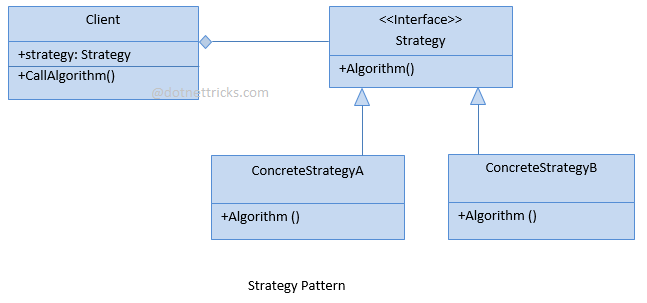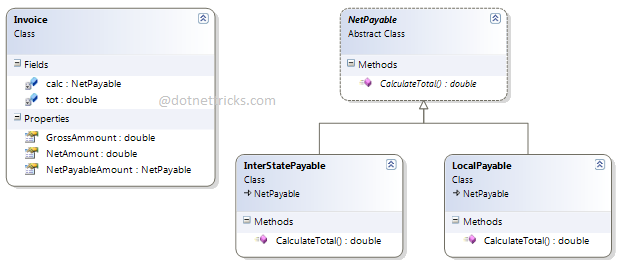# Strategy Design Pattern - C#

22 Aug 2022
Intermediate
54.2K Views

Strategy Design Pattern falls under Behavioral Pattern of Gang of Four (GOF) Design Patterns in .Net. The command pattern is commonly used in the menu systems of many applications such as Editor, IDE, etc. In this article, I would like to share what is strategy pattern and how is it work?

## What is Strategy Design pattern?

This pattern allows a client to choose an algorithm from a family of algorithms at run-time and gives it a simple way to access it.

Strategy Design Pattern involves the removal of an algorithm from its host class and putting it in a separate class. As you know, there may be multiple strategies which are applicable for a given problem. So, if the algorithms will exist in the host class, then it will result in a messy code with lots of conditional statements.

## Strategy Design Pattern - UML Diagram & Implementation

The UML class diagram for the implementation of the Strategy Design Pattern is given below:The classes, interfaces, and objects in the above UML class diagram are as follows:

1. ### Context

This is a class that contains a property to hold the reference of a Strategy object. This property will be set at run-time according to the algorithm that is required.

2. ### Strategy

This is an interface that is used by the Context object to call the algorithm defined by a ConcreteStrategy.

3. ### ConcreteStrategyA/B

These are classes that implement the Strategy interface.

### C# - Implementation Code

```public class Client
{
public IStrategy Strategy { get; set; }

public void CallAlgorithm()
{
Console.WriteLine(Strategy.Algorithm());
}
}

public interface IStrategy
{
string Algorithm();
}

public class ConcreteStrategyA : IStrategy
{
public string Algorithm()
{
return "Concrete Strategy A";
}
}

public class ConcreteStrategyB : IStrategy
{
public string Algorithm()
{
return "Concrete Strategy B";
}
}

```

## Real Life Example:## When to use it?

1. There are multiple strategies for a given problem and the selection criteria of a strategy are defined as a run-time.

2. Many related classes only differ in their behaviors.

3. The strategies use the data to which the client has no access.

Read More Articles Related to Design patterns